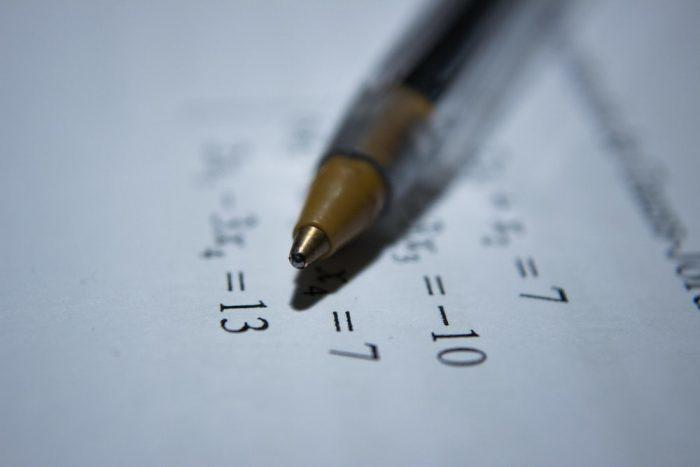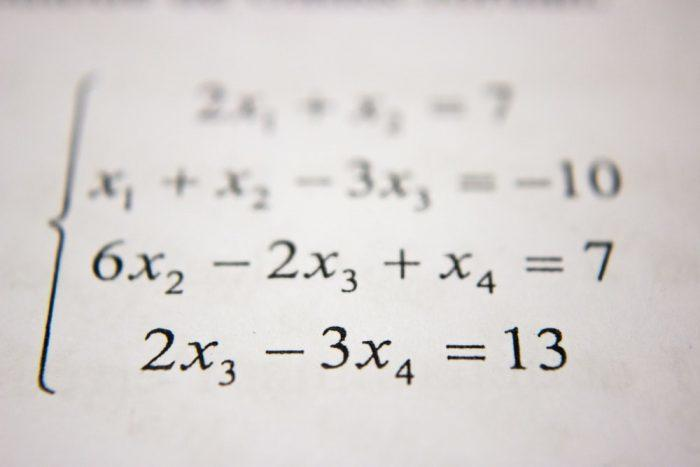The ACT Math test might seem overwhelming for those who lack pre-algebra, algebra, and geometry experience.

Also, if it's been too long since you've picked up a calculator or looked at a complex math problem, remembering formulas and theorems might seem hard.

Most students who take the ACT test find the Math section the most challenging part. But it doesn't always have to be a struggle.

With a decent ACT Math study guide, a qualified tutor, and a proper understanding of the concepts at play, the ACT Math section will be a walk in the park!

Fortunately, the ACT Math section prioritizes data analysis over raw calculations, meaning that you will have to deduce answers from analyzing sets of data like algebraic expressions rather than scrunching down numbers in long equations.

To help you succeed, we will discuss the essential concepts to help you score well on the ACT math exam. Keep in mind that this list is not exhaustive, and it might not cover all the topics that appear on the ACT exam.

We listed the topics students struggle with the most and regularly feature in the exam.

Any decent ACT math tutor will tell you to focus on the topics' fundamentals instead of cramming formulas and memorizing everything.

Therefore, it's essential to define each math concept and break down the workings step by step, so let's start:The best Mathematics tutors available5 (51 reviews)
Davayne
\$13
/h1st lesson free!5 (25 reviews)
Jeffry
\$50
/h1st lesson free!4.9 (9 reviews)
Lyle
\$25
/h1st lesson free!5 (5 reviews)
Bryan
\$25
/h1st lesson free!5 (4 reviews)
Beccalynn
\$18
/h1st lesson free!5 (79 reviews)
Dr. Mary (professional expert tutor)
\$50
/h1st lesson free!5 (9 reviews)
Anh
\$25
/h1st lesson free!4.9 (10 reviews)
Momin khan
\$10
/h1st lesson free!5 (51 reviews)
Davayne
\$13
/h1st lesson free!5 (25 reviews)
Jeffry
\$50
/h1st lesson free!4.9 (9 reviews)
Lyle
\$25
/h1st lesson free!5 (5 reviews)
Bryan
\$25
/h1st lesson free!5 (4 reviews)
Beccalynn
\$18
/h1st lesson free!5 (79 reviews)
Dr. Mary (professional expert tutor)
\$50
/h1st lesson free!5 (9 reviews)
Anh
\$25
/h1st lesson free!4.9 (10 reviews)
Momin khan
\$10
/h1st lesson free!

## 5 Major Topics In The ACT Math Test

The ACT math test aims to test your arithmetic capabilities to ensure that you're a good fit for any future academic degree you may enroll in.

### 1. Pre-Algebra

The Pre-Algebra section constitutes a significant chunk of the math ACT exam, accounting for 20-25% of the test. This section will cover simple topics like probability, mean, median, and mode.

Mean, median, and mode are relatively simple concepts to grasp, but it's still wise to know the difference between them.

Mean denotes the average of all numbers in a set, the median is the middle number in a range of numbers and mode is the most occurring number.

The second sub-topic in pre-algebra is probability. It's a straightforward concept that involves dividing the number of specific outcomes by the total number of outcomes to get an event's likelihood.

Are you flipping a coin? You have a 1 in 2 probability of getting heads!

Another sub-topic you will cover in the pre-algebra ACT exam is absolute value. If you see vertical lines around a number, you need to treat it like a positive number to solve in an equation.

That's really what an absolute value is all about.

### 2. Elementary Algebra

As we progress to elementary algebra, things get slightly more complex, but nothing you are likely to find challenging.

Fortunately, it forms a lesser chunk of the test, at around 15-20%, compared to the former section.

It will teach you to form algebraic expressions and construct word problems into equations with variables.

It's essential to understand how to pinpoint critical information in the problem to master this concept.

In this section, you will also learn how to multiply binomials. It's an easy topic, and you might probably already have heard of the FOIL technique or the first-outer-inner-last technique.

All you need is a little practice to perfect this technique, especially if you're dealing with negative numbers.

It may benefit you to take a few ACT math practice tests to get a feel for the questions.

Lastly, you will have to work on inequalities. By inequalities, we mean the math concept, with the greater than and less than signs.

All you need to do is treat them like any other equation and switch the inequality signs when you multiply or divide by a negative number.Algebra forms a hefty portion of the ACT test syllabus, so you want to spend enough time perfecting this topic. However, it would help if you tried to time yourself to ensure that you don't waste your time on one question. (Source: Pixabay)

### 3. Intermediate Algebra

This section also makes up around 15 to 20% of the ACT math exam, and this is where you get to deal with more complex algebraic concepts, such as functions and logarithms.

Candidates will deal with functions and the relationships between the sides of an equation, including multiplication and division.

If you're multiplying both sides, the two sides will go up, but the two sides will have an inverse relationship if you're dividing them.

When dealing with functions, you will learn how plugging a variable into f(x) is no different from plugging a variable in any equation.

You will also deal with compound functions like f(g(x)), where you will have to plug one function into another function.

Lastly, you'll be dealing with logarithms, but the good news is that they very rarely feature on the ACT.

It's still a good idea to include them in your ACT practice test, though. If you're aware of exponents, logarithms are just the reverse of exponential functions.

The concept may sound a little complex, but once you go through a few practice problems, it's surprisingly easy to grasp.

### 4. Coordinate Geometry

That brings us to our second-last section, which makes up a hefty 20 to 25% of the ACT math exam. This is the coordinate geometry section, where you'll be dealing with conic sections and equations of a line, among other topics.

Talking about conic sections, here you'll learn of parabolas, ellipses, and circles. They might seem similar, but they're different shapes with different curves and equations.

A parabola is the visual representation of the quadratic formula and usually looks like a U-shaped curve, curving upward or downwards.

Circles are pretty obvious shapes, and it's likely you already have the fundamental knowledge about circular geometry, such as measuring circumferences and radii, as well as the area of a circle.

Moreover, ellipses are pretty similar to circles, but it's necessary to pay attention to the center of the ellipse, denoted by the values h and k.

We're confident you know the equations of a line, specifically the equation y = MX + b, where M is the slope and b is the y-intercept.

Moving on from there, you will find different equations centered around this concept that can be simplified to this basic equation.

You will also deal with simple three-dimensional geometry in your ACT math study guide. Here you will have to calculate the volume and surface area of solids like cubes and prism.

To make it easy for you, here are a few pointers:

• When calculating a rectangular solid's surface area, multiply the length by width and multiply this answer by two for the solid's end sides. Then multiply the length and width for the longer sides and multiply this number by four. Now add up the longer sides and end sides to get the total surface area.
• Dealing with volume is also easy. All you must do is multiply the length, width, and height of the solid. You might need to find the diagonal length, which can be found by adding the square of the width, length, and height and then taking the root of the total number.It's imperative to know how to manage your time on the ACT Math test if you want to answer every question. Managing your time requires more practice than the actual test material itself, which is why regular practice is essential.  (Source: Unsplash)

### 5. Trigonometry

That brings us to our last topic, which makes up only 5 to 10% of the math ACT test, but is still important nonetheless. This section covers trigonometry, where you'll learn about sine, cosine, and tangents.

These terms are related to the sides of a triangle, and a great way to remember them is through a mnemonic device called the SOHCAHTOA.

The SOH refers to the sine value and involves the opposite over the hypotenuse. The CAH denotes the cosine and consists of the adjacent over the hypotenuses, while the TOA involves the sine over the cosine.

Once acquainted with sine, cosine, and tangents, you will be asked to find unknown lengths of one of the triangle's sides, using angles of 0, 30, 45, and 90.

For this, you will need to memorize the sine, cosine, and tangent values for these angles, but they're simple.  We recommend using flashcards to make it easy to remember these values.

Lastly, you'll be learning about trigonometric graphs, namely the graphs of sin x, cos x, and tan x. Once you know how these graphs look like, it will be easy to match them to equations, even if the graph's amplitude and period have been modified.Your tutor will be making you attempt ACT math practice tests to get you accustomed to the type of problems you will be solving. This experience is invaluable as it will prepare you for the challenges that lay ahead. (Source: Unsplash)

## Learning ACT Math With Superprof

If you're still stressing out over your exam and the ACT test dates are close, make life simpler for yourself, and sign up for private ACT math lessons with Superprof.

Search for highly qualified ACT tutors around you and get started with your math lessons right away.The platform that connects tutors and students5.00 (1 rating(s))Loading...